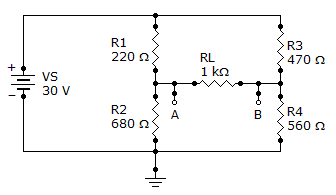# Electrical Engineering - Circuit Theorems and Conversions - Discussion

### Discussion :: Circuit Theorems and Conversions - General Questions (Q.No.11)

11.

What is the Thevenin equivalent (VTH and RTH) for the circuit given?[A]. 6.4 V, 422[B]. 6.4 V, 560[C]. 6.4 V, 680[D]. 30 V, 422Explanation:

No answer description available for this question.

 M.V.Krishna/Palvoncha said: (Dec 19, 2011) From the circuit Vth= ((R1||R3)*Vs)/(R1+R3); (note: R1||R3 means R1 in parllel with R3, R1||R3=149.8); on sloving we get Vth=(149.8*30)/690 Vth=6.5 (app. equal to 6.4v; from the circuit we get Rth=(R1+R3)||(R2+R4) Rth=690||1240; Rth=443.31(app. equal to 422 ohm) so option A

 Praveen Juturu said: (Dec 9, 2013) Thevenin's resistance is (R1 // R2) + ( R3 // R4). Thevenin's voltage is obtained by applying potential divider rule, Vth = Voltage across R2 - voltage across R4.

 Subarna Das said: (Jul 2, 2014) Vth = {30*(470/470+560)}-{30*(220/220+680)}. Vth = 6.3V. Rth = (470+220)//(680+560). Rth = 443 ohm. So, option 1 is right.

 Shashi said: (Jan 10, 2015) R1 parallel to R2& R3 is parallel to R4 and the result of R1||R2&R3||R4 are in series. = 220||680+470||560 = 421.75 (422).

 Naveedabanu said: (May 26, 2015) I agree with answers. But I am not getting series and parallel combination. Please tell me if you know.

 Nami said: (Nov 1, 2015) What about vth then?

 Aswathy said: (Jul 23, 2016) Rth = (R1 // R2) + ( R3 // R4). = 220||680 + 470||560 = 421.75 (422). Vth is the open circuited voltage therefore find the effective resistance neglecting RL R eff = R1 + R2 II R3 + R4. = 220 + 680 II 560 + 470 = 900 II 1030= (900 * 1030) /(900 + 1030) = 480.31. Now find the total current. I = V/Reff =30/480.31 = 0.062. Find current through each parallel branch using current division: I1 = (0.062 * 1030)/(1030 + 900) = 0.033. I2 = 0.062 - 0.033 = 0.029. Vth= VA - VB = V470 - V220. =(470 * 0.029) - (220 * 0.033) = 6.37 v.

 Akhila said: (Jul 26, 2016) Well said, Thank you all.

 Shan said: (Jan 6, 2017) Vth is 6.28. Use vlt divider rule.

 Prashant said: (Aug 9, 2017) Thanks @Aswathy.

 Pkgp said: (Dec 13, 2018) Thank you all.

 Praveen said: (Jun 19, 2019) Thanks for explaining it.